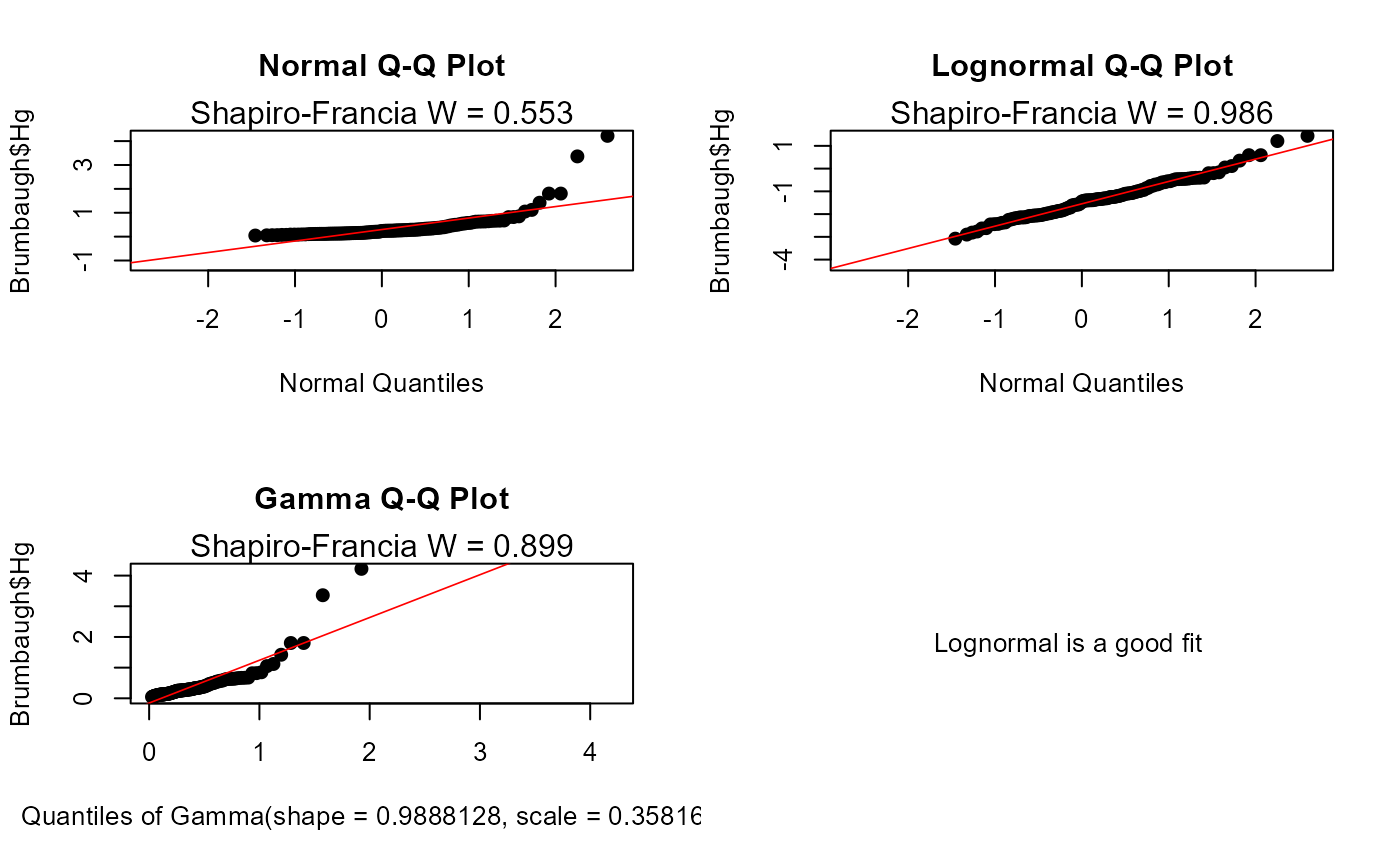Produces three quantile-quantile (Q-Q) plots, also called probability plots, based on three distributions (normal, lognormal and gamma distributions).

cenCompareQQ(x.var, cens.var, Yname = yname, printrslt = TRUE, ...)

## Arguments

x.var

The column of x (response variable) values plus detection limits

cens.var

The column of indicators, where 1 (or TRUE) indicates a detection limit in the y.var column, and 0 (or FALSE) indicates a detected value in y.var.

Yname

Optional – input text in quotes to be used as the variable name on all plots. The default Yname is the name of the y.var input variable.

printrslt

Logical TRUE/FALSE option of whether to print the best distribution in the console window, or not. Default is TRUE.

...

further graphical parameters (from par), such as srt, family and xpd.

## Value

Plots three Q-Q plots based on normal, lognormal and gamma distributions and prints the best-fit distribution.

## Details

Produces three Q-Q plots and reports which one has the highest Shapiro-Francia test statistic (W). The distribution with the highest W is the best fit of the three.

## Examples


data(Brumbaugh)
cenCompareQQ(Brumbaugh$Hg,Brumbaugh$HgCen)
#> Lognormal is a good fit